GATE  >  GATE Numerical Ability Questions (Quant & Analytical) -2

# GATE Numerical Ability Questions (Quant & Analytical) -2

Test Description

## 10 Questions MCQ Test General Aptitude for GATE | GATE Numerical Ability Questions (Quant & Analytical) -2

GATE Numerical Ability Questions (Quant & Analytical) -2 for GATE 2023 is part of General Aptitude for GATE preparation. The GATE Numerical Ability Questions (Quant & Analytical) -2 questions and answers have been prepared according to the GATE exam syllabus.The GATE Numerical Ability Questions (Quant & Analytical) -2 MCQs are made for GATE 2023 Exam. Find important definitions, questions, notes, meanings, examples, exercises, MCQs and online tests for GATE Numerical Ability Questions (Quant & Analytical) -2 below.
Solutions of GATE Numerical Ability Questions (Quant & Analytical) -2 questions in English are available as part of our General Aptitude for GATE for GATE & GATE Numerical Ability Questions (Quant & Analytical) -2 solutions in Hindi for General Aptitude for GATE course. Download more important topics, notes, lectures and mock test series for GATE Exam by signing up for free. Attempt GATE Numerical Ability Questions (Quant & Analytical) -2 | 10 questions in 15 minutes | Mock test for GATE preparation | Free important questions MCQ to study General Aptitude for GATE for GATE Exam | Download free PDF with solutions
 1 Crore+ students have signed up on EduRev. Have you?
GATE Numerical Ability Questions (Quant & Analytical) -2 - Question 1

### Select the graph that schematically represents BOTH y = xm and y = x1/m properly in the interval 0 ≤ x ≤ 1, for integer values of m, where m > 1.

Detailed Solution for GATE Numerical Ability Questions (Quant & Analytical) -2 - Question 1

Given y = xm where m > 1 & y = x1/m and 0 ≤ x ≤ 1

Let us consider m = 2

In that case:

y = x2 graph can be plotted as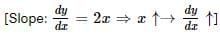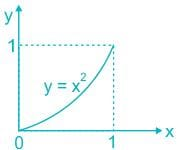Also y = x0.5 is represented in graph as: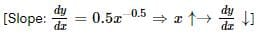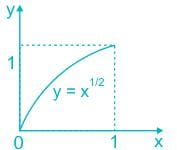∴ y = xm & y = x1/m is correctly represented by option 1) i.e.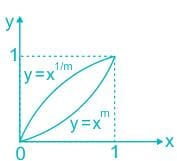y = xm ⇒ increasing slope

y = x1/m ⇒ decreasing slope

GATE Numerical Ability Questions (Quant & Analytical) -2 - Question 2

### It was estimated that 52 men can complete a strip in a newly constructed highway connecting cities P and Q in 10 days. Due to an emergency, 12 men were sent to another project. How many numbers of days, more than the original estimate, will be required to complete the strip?

Detailed Solution for GATE Numerical Ability Questions (Quant & Analytical) -2 - Question 2

Concept:

M× D= M× D2

Where,

M1 = Number of men in initial condition, M2 = Number of men in final condition

D1 = Number of days required in the initial condition,

D2 = Number of days required in the final condition

Calculation:

Given:

M= 52,

D= 10, M= 52 - 12 = 40,

D= ?

52 × 10 = 40 × D­2

D= 13 days

Now,

Numbers of days more than the original estimate is equal to:

Number of days required in the final condition - Number of days required in the initial condition

∴ Numbers of days more than the original estimate = D2  D1

∴ Numbers of days more than the original estimate = 13 – 10

∴ Numbers of days more than the original estimate = 3 days

GATE Numerical Ability Questions (Quant & Analytical) -2 - Question 3

### M and N had four children P, Q, R and S. Of them, only P and R were married. They had children X and Y respectively. If Y is a legitimate child of W, which one of the following statements is necessarily FALSE?

Detailed Solution for GATE Numerical Ability Questions (Quant & Analytical) -2 - Question 3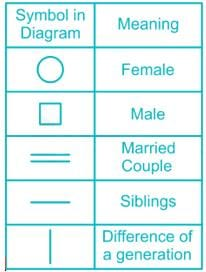Using the above symbols we get the following family tree: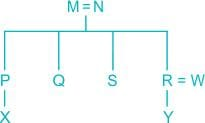From the tree diagram, we can see that R and W are the parents of Y as Y is a legitimate child of W.

Therefore it is possible that R is the father of Y and W is the wife of R. Hence the statements in options 2 and 3 are not necessarily false.

Also, from the tree diagram, we can conclude that M and N are the grandparents of Y and hence the statement in option 1 is not necessarily false.

As Y is a legitimate child of W, W is the spouse of R and not P.

Hence the statement 'W is the wife of P' is necessarily false.

GATE Numerical Ability Questions (Quant & Analytical) -2 - Question 4

There are five levels {P, Q, R, S, T} in a linear supply chain before a product reaches customers, as shown in the figure.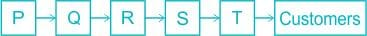At each of the five levels, the price of the product is increased by 25%. If the product is produced at level P at the cost of Rs. 120 per unit, what is the price paid (in rupees) by the customers?

Detailed Solution for GATE Numerical Ability Questions (Quant & Analytical) -2 - Question 4

Concept:

Price paid by customer at end = initial cost × (increased percentage)n

Where,

n = no. of levels

Calculation:

Given:

Cost of product at first level P = 120 Rupees.

Also,

It is increased by 25% in each level.

Hence,

Initial cost = 120 Rupees.,

Increased percentage = 25%,

n = 5

Now,

Price paid by customer at end = 120 × 1.255 Rupees

∴ Price paid by customer at end = 366.21 Rupees.

GATE Numerical Ability Questions (Quant & Analytical) -2 - Question 5

Given that a and b are integers and a + a2 b3 is odd, which one of the following statements is correct?

Detailed Solution for GATE Numerical Ability Questions (Quant & Analytical) -2 - Question 5

Given (a + a2b3) is odd

⇒ a (1 + ab3) is odd

As the multiplication of two numbers is odd only when those two numbers are odd.

That means ‘a’ is odd and (1 + ab3) is also odd

⇒ ab3 is even

As ‘a’ is an odd number, b3 should be even number to get ab3 as even.

bis even only when b is even.

Therefore, ‘a’ is odd and ‘b’ is even.

GATE Numerical Ability Questions (Quant & Analytical) -2 - Question 6

The bar graph shows the data of the students who appeared and passed in an examination for four school P, Q, R and S. The average of success rates (in percentage) of these four schools is _____.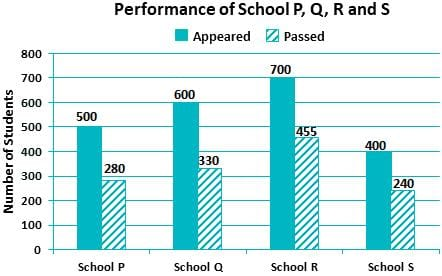Detailed Solution for GATE Numerical Ability Questions (Quant & Analytical) -2 - Question 6

Concept:

Average success rate of one school = Total no of passed students/Total no. of students who appeared

The average success rates of these four schools = Sum of Average success rate of all four schools/Total no. of schools

Therefore, The average success rates of these four schools will be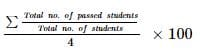Calculation:

The average success rates of these four schools will be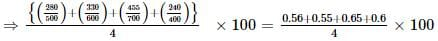= 59%

∴ The average success rates of these four schools = 59.0%

GATE Numerical Ability Questions (Quant & Analytical) -2 - Question 7

A person divided an amount of Rs. 100,000 into two parts and invested in two different schemes. In one he got 10% profit and in the other he got 12%. If the profit percentages are interchanged with these investments he would have got Rs.120 less. Find the ratio between his investments in the two schemes.

Detailed Solution for GATE Numerical Ability Questions (Quant & Analytical) -2 - Question 7

Let Rs. 100,000 is divided into two parts x and y respectively.

Part I: Scheme – 1 (10% profit)

After profit in Scheme – 1, he will get 1.10x rupees

Scheme – 2 (12% profit)

After profit in Scheme – 2, he will get 1.12y rupees

Total amount that he will get from Scheme 1 and Scheme 2 after applying profits of 10% and 12% respectively = 1.10x + 1.12y     …1)

Part II: When profit percentages are interchanged

Scheme – 1: (12% profit), Scheme – 2: (10% profit)

Total amount after applying these profit percentages = 1.12x + 1.10y     …2)

Given that,

1.10x + 1.12y – 1.12x – 1.10y = 120

0.02y – 0.02x = 120

2y – 2x = 12000

y – x = 6000     …3)

y + x = 100000     …4)

Solving 3) and 4)

2y = 106000

Y = 53000, x = 47000

Ratio between the investments = x/y = 47/53

GATE Numerical Ability Questions (Quant & Analytical) -2 - Question 8

Consider the following three statements:

(i) Some roses are red.

(ii) All red flowers fade quickly.

Q. Which of the following statements can be logically inferred from the above statements?

Detailed Solution for GATE Numerical Ability Questions (Quant & Analytical) -2 - Question 8

Consider the following Venn diagram: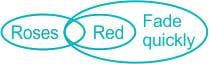If all red flowers fade quickly and some roses are red, then these roses being red flowers will also fade quickly.

Hence, it can be inferred that some rose fade quickly if the statements (i) and (ii) are true.

Whereas if any of the statements (i) and (ii) are false, nothing can be inferred about statement (iii).

GATE Numerical Ability Questions (Quant & Analytical) -2 - Question 9

A rectangle becomes a square when its length and breadth are reduced by 10 m and 5 m, respectively. During this process, the rectangle loses 650 m2 of area. What is the area of the original rectangle in square meters?

Detailed Solution for GATE Numerical Ability Questions (Quant & Analytical) -2 - Question 9

Let the length and breadth of the rectangle is L and B respectively.

The area of the rectangle = LB

It becomes square when its length and breadth are reduced by 10 m and 5 m, respectively.

Now, the length and breadth of the square become (L – 10) and (B – 5) respectively.

As in a square the length and breadth are the same, L – 10 = B – 5

⇒ L = B + 5

The area of the square = (L – 10) (B – 5)

Given that, the rectangle loses 650 m2 of area

⇒ LB – 650 = (L – 10) (B – 5)

⇒ LB – 650 = LB + 50 – 10B – 5L

⇒ 10B + 5L = 700

⇒ 10B + 5(B + 5) = 700

⇒ B = 45 m

⇒ L = B + 5 = 50 m

Area of the original rectangle = LB = 50 × 45 = 2250 m2

GATE Numerical Ability Questions (Quant & Analytical) -2 - Question 10

The value of the expression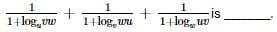Detailed Solution for GATE Numerical Ability Questions (Quant & Analytical) -2 - Question 10

Concept:

Logarithmic Properties: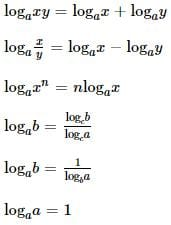Calculation: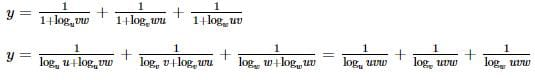Using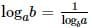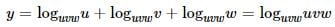= 1

## General Aptitude for GATE

73 videos|86 docs|108 tests
 Use Code STAYHOME200 and get INR 200 additional OFF Use Coupon Code
Information about GATE Numerical Ability Questions (Quant & Analytical) -2 Page
In this test you can find the Exam questions for GATE Numerical Ability Questions (Quant & Analytical) -2 solved & explained in the simplest way possible. Besides giving Questions and answers for GATE Numerical Ability Questions (Quant & Analytical) -2, EduRev gives you an ample number of Online tests for practice

## General Aptitude for GATE

73 videos|86 docs|108 tests